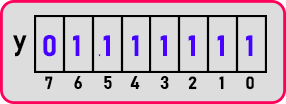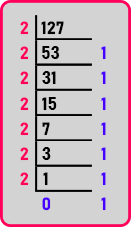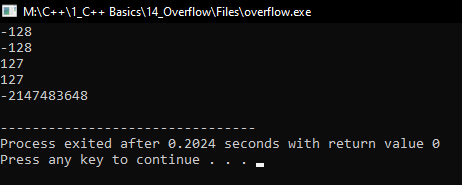# Overflow in C++

## Overflow in C++ with Examples

In this article, I am going to discuss Overflow in C++ with Examples. Please read our previous article where we discussed Increment Decrement Operator in C++ with Examples.

##### Overflow in C++:

This is a concept and very useful concept for interviews. In interviews they may ask questions directly upon overflow or questions they may frame depending on overflow. It is a general topic not just related to C++. This concept applies to every programming language. Before understanding overflow, we have to revise a few concepts that we have learned in previous articles. For that, we have taken an example here,

char x = 127;

We have a character type variable ‘x’ and have value 127. How many bytes do the characters take? It takes 1 byte, 1 byte = 8 bits.So, this is one bite and we are showing all the bits that are 0 to 7. 0th bit is the least significant bit and the 7th bit is the most significant, then how data is actually represented in memory. It is represented in binary form. So, this number of 127 is stored in binary forms, so how it looks like in binary form. This is the conversion from a decimal number to a binary number.We have already learned this so I have got the digits as ‘111111’, seven ones. The 7th bit is the sign bit. If it is 0 means the number is positive. If it is 1 means the number is negative. Then one more thing we should know what is the range of values that a character can store. The range of values is from -128 to 127. Now coming to the next concept.

char x = 127;

The variable is having the largest value possible which is the maximum value. Beyond that, we cannot have any value in the character type variable. So right now, it is 127, I will make it as ‘++x’. If I write this statement then what it will become. It will try to go to the next value which is not there.

##### So, what happens to the data?

This will become -128 when we will try to cross this value beyond this one, so it will come down to this one and vice versa. If it is -128 and if we try to reduce it decrease it by 1 then it will go to 127. So, it’s more like cyclic. This cyclic behavior is called overflow. So, when the value is more than the capacity, it will take the values again from the beginning that is overflow.

##### Why does it happen?

Why it happens and how magical it takes the values that I will show you here. Suppose, in binary, we have the value ‘1111111’ and already I have a number here. Now in this binary let us add 1 because ‘++’ means it will increase by 1.

So, if it is increased by 1 if one is added to ‘1111111’ then what will be the result? It will be ‘10000000’ means the most significant bit or sign bit will be changed and all the previous values will change to zero and if the sign bit is on, then we shift to negative or positive.

But what is the value? The value is 128. See the value is 128 and also the same bit does act as a sign bit. Now one more thing actually the number that we got is in two’s complement because negative numbers are stored in two’s complement. So, if I want to get the original number then again, we should find out its two’s complement but, in this case, I get back the same number you can do it by yourself. So, find two’s complement you’ll get back the same thing again. So let’s see this in the code part:

##### Overflow Code in C++ Language:
```#include <iostream>
using namespace std;
int main()
{
char a = 128;
cout << (int) a << endl;
char b = 127;
b++;
cout << (int) b << endl;
char c = -129;
cout << (int) c << endl;
char d = -128;
d--;
cout << (int) d << endl;
int e = INT_MAX;
e++;
cout << (int) e << endl;
return 0;
}
```
###### Output:In the next article, I am going to discuss Bitwise Operators in C++ with Examples. Here, in this article, I try to explain Overflow in C++ with Examples and I hope you enjoy this Overflow in C++ with Examples article.

### 1 thought on “Overflow in C++”

1.This program thows error. char to int converstion